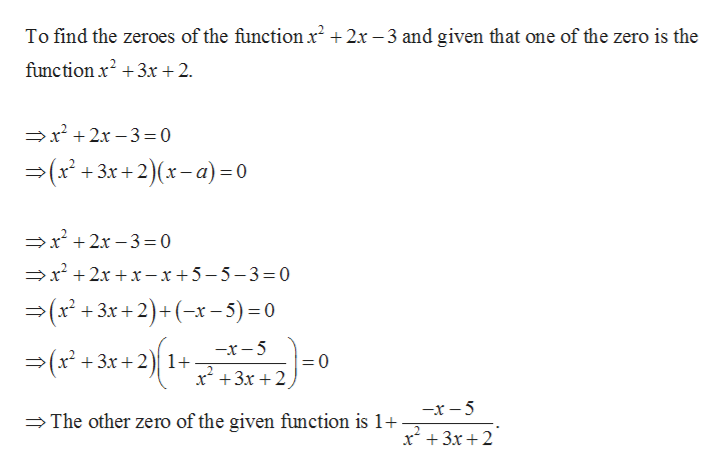What are the zeros of the function x^2+2x-3 over x^2+3x+2

Question

What are the zeros of the function x^2+2x-3 over x^2+3x+2

Step 1

To find the zeroes of the function x2+2x-3 over x2+3x+2.

The zeroes of a function are the values such that substituting those values in the function will results in zero. The zeroes of a function over another function means that one factor is the given function.

Step 2

Find the zero of the function over t...help_outlineImage TranscriptioncloseTo find the zeroes of the functionr2+2x -3 and given that one of the zero is the function x23x + 2 x2x-3 0 (r+3 x+2)(x-a)=0 2x-3=0 r2xr -x+5-5-3= 0 — (x* + 3к +2) +(-*-5) -0 —х — 5 Зх + 2)| 1+ =0 2 x 3x2 —х — 5 The other zero of the given function is 1+ x3x2 fullscreen

Want to see the full answer?

See Solution

Want to see this answer and more?

Our solutions are written by experts, many with advanced degrees, and available 24/7

See Solution
Tagged in

Polynomials